Small Bore Orifice Flow Meter Calculation for Gas Flow

For pipe diameter < 5 cm. Compute flow rate, bore diameter, or differential pressure. Equations: ASME MFC-14M-2001

Register to enable "Calculate" button.

 Mass Flow, M (kg/s): Actual Vol Flow, Qa (m3/s): Solve for: Std Vol Flow, Qs (std m3/s): Flow Rate Differential Pressure. (M known) Differential Pressure. (Qa known) Differential Pressure. (Qs known) Throat Diameter. (M known) Throat Diameter. (Qa known) Throat Diameter. (Qs known) Upstream Abs Pressure, P1 (Pa): Differential Pressure, Δp (Pa): Select Meter Type and Gas: Pressure Ratio, P2/P1: Air (enter P1, T) Carbon dioxide (enter P1, T) Hydrogen (enter P1, T) Methane, Natural Gas (enter P1, T) Nitrogen (enter P1, T) Oxygen (enter P1, T) User enters P1, density, viscosity, K Throat Diameter, d (m): Corner Pressure Taps Flange Pressure Taps Pipe Diameter, D (m): Ratio, d/D: Select Units for each Variable: Gas Temperature, T (K): Mass Flow in slug/s Mass Flow in lb (mass)/s Mass Flow in lb (mass)/hr Mass Flow in kg/s Density (kg/m3): Qa and Qs in ft3/s or cfs Qa and Qs in ft3/min or cfm Qa and Qs in ft3/hr Qa and Qs in ft3/day Qa and Qs in m3/s Qa and Qs in m3/min Qa and Qs in m3/hr Qa and Qs in m3/day Dynamic Viscosity (N-s/m2): P1 in psi P1 in inch H2O (60F) P1 in inch Mercury (60F) P1 in millibars P1 in N/m2 or Pa P1 in mm Mercury (0C) Isentropic Exponent, K: Diff P and P Loss in psi Diff P and P Loss in inch H2O (60F) Diff P, P Loss in inch Mercury (60F) Diff P and P Loss in millibars Diff P and P Loss in N/m2 or Pa Diff P and P Loss in mm Mercury (0C) Gas Expansibility, e: Diameters in inches Diameters in feet Diameters in cm Diameters in meters Discharge Coefficient, C: Temperature in Fahrenheit, F Temperature in Rankine, R Temperature in Celcius, C Temperature in Kelvin, K Reynolds No. based on d, Red: Density in slug/ft3 Density in lb(mass)/ft3 Density in kg/m3 Density in g/cm3 Reynolds No. based on D, ReD: Viscosity in lb-s/ft2, slug/ft-s Viscosity in N-s/m2, kg/m-s Viscosity in poise, P Viscosity in centiPoise, cP Pressure Loss, w (Pa): Velocity in ft/s; Area in ft2 Velocity in m/s; Area in m2 Minor Loss Coefficient, Km: © 2014 LMNO Engineering, Throat Velocity, Vthroat (m/s): Research, and Software, Ltd. Pipe Velocity, Vpipe (m/s): http://www.LMNOeng.com Throat Area, Athroat (m2): Pipe Area, Apipe (m2):

Units in small bore orifice for gas flow calculation: C=degrees Celsius, cm=centimeter, cP=centipoise, cSt=centistoke, F=degrees Fahrenheit, cfm=cubic feet per minute, cfs=cubic feet per second, ft=foot, g=gram, hr=hour, in=inch, K=degrees Kelvin, kg=kilogram, lb=pound, m=meters, mbar=millibar, min=minute, mm=millimeter, N=Newton, Pa=Pascal, psi=pound per square inch, R=degrees Rankine, s=second, scfm=standard cfm, std=standard.

Types of Pressure Taps for Small Bore Orifices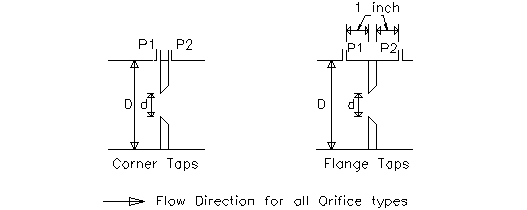Introduction to Small Bore Orifice for Gas Flow Calculation
Orifice flow meters are used to determine a liquid or gas flow rate by measuring the differential pressure P1-P2 across the orifice plate. Orifice flow meters are generally less expensive to install and manufacture than the other commonly used differential pressure flow meters; however, nozzle and venturi flow meters have the advantage of lower pressure drops.

The orifice calculation on this page is for flow of gases. Please see the links at the top of this page for liquid flow through orifice meters. Gas flow calculations include an expansibility factor e, which is not present in the liquid calculation. The expansibility factor accounts for the effect of pressure change on gas density as gas flows through the orifice. Our calculation is valid for subsonic gas flow.

An orifice flow meter is typically installed between flanges connecting two pipe sections (flanges are not shown in the above drawings). The two standard pressure tapping arrangements are shown in the drawings; the location of the pressure taps affects the discharge coefficient somewhat. Flange pressure taps penetrate the flange and are at a standard distance of 1 inch (2.54 cm) from either side of the orifice. For corner taps, the pressure tap locations are as shown. For exact geometry and specifications for orifices, see ASME (2001).

Equations for Small Bore Orifice for Gas Flow                             Top of Page
The calculations on this page are for orifices carrying a gas as described in ASME (2001).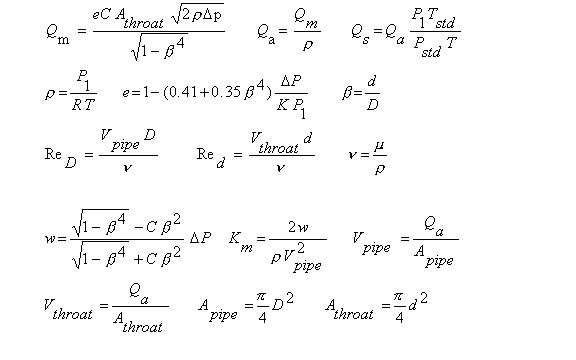Discharge Coefficients for Small Bore Orifice for Gas Flow (ASME, 2001)               To top of page
Corner Taps: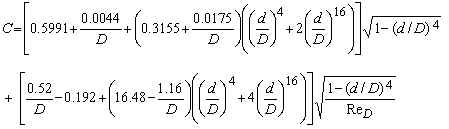Flange Taps: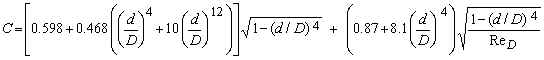where D is in inches; and d/D, ReD, and C are dimensionless.

Validity of Small Bore Orifice Calculation for Gas Flow (ASME, 2001)                             Top of Page
Pipe Diameter D
LMNO Engineering orifice calculation requires 0.635 cm ≤ D ≤ 5.08 cm for both corner and flange taps.
ASME (2001) suggests 1.2 cm ≤ D ≤ 4 cm for corner taps and 2.5 ≤ D ≤ 4 cm for flange taps.

Diameter ratio d/D
LMNO Engineering and ASME (2001) require 0.1 ≤ d/D ≤ 0.8 for corner taps and 0.15 ≤ d/D ≤ 0.7 for flange taps.

Reynolds number based on pipe diameter ReD
LMNO Engineering and ASME (2001) require ReD ≥ 1000.

Expansibility e
The orifice equation shown above for expansibility e is valid for P2/P1 ≥ 0.8. Our orifice calculation gives a warning message if P2/P1 < 0.8, but still computes answers.

Built-in Properties for Certain Gases
To provide ease of use, our orifice calculation has properties of some gases built-in to the calculation. The user can select Air, Carbon dioxide, Hydrogen, Methane (natural gas), Nitrogen, or Oxygen. The density is automatically computed using the ideal gas law based on the upstream pressure and temperature entered. The dynamic viscosity is a function of temperature and uses the methodology shown on our Gas Viscosity page. The isentropic exponent, K, is based on the specific heat ratio. For methane, the dynamic viscosity value shown in the orifice calculation is valid for 0 oF ≤ T ≤ 1000 oF. If T<0 oF, then the viscosity value shown and used in the orifice computation is the viscosity at 0 oF. If T>1000 oF, then the viscosity value shown and used in the orifice computation is the viscosity at 1000 oF (0 oF is -17.8 oC and 1000 oF is 537.8 oC). For all other gases shown in the drop-down menu, there is no temperature limitation on the validity of the viscosity. Dynamic viscosity is essentially independent of pressure.

If you know that your density, viscosity, or isentropic exponent is significantly different than the value shown in the orifice calculation, then you can select "User enters P1, density, viscosity, K" and enter these values manually. Also, if the gas is not listed in our drop-down menu, then you can select "User enters P1, density, viscosity, K" and enter these values manually. K must be > 1. Additionally, values for K can be found in Weast (1985, p. F-11), Perry and Green (1984, p. 3-144), and other sources.

Pressure Loss
w is the static pressure loss occurring from a distance of approximately D upstream of the orifice to a distance of approximately 6D downstream of the orifice. It is not the same as differential pressure. Differential pressure is measured at the exact locations specified in ASME (2001) (shown in the above figures).

Minor Loss Coefficient
Km is computed to allow you to design pipe systems with orifices and incorporate their head loss. Head loss is computed as h=KmV2pipe/2g.

Standard Volumetric Flow Rate
Standard volumetric flow rate, Qs, is the volumetric flow rate computed at standard pressure and temperature, Pstd and Tstd (shown above in variables). Actual flow rate, Qa, is computed at the gas's actual pressure and temperature. Qs is useful to users who need to compute (or input) standard flow rate; often pump curves and flow measurement devices provide standard, rather than actual, flow rate. The advantage of using standard flow instead of actual flow is that the same device (or pump curve) can be used for a gas at various temperatures and pressures without re-calibrating for an infinite range of actual pressures and temperatures. The user can easily convert standard to actual flow rate if the actual temperature and pressure of the gas are known; our orifice calculation does this automatically.

Variables in Small Bore Orifice for Gas Equations:                             Top of Page
Dimensions: F=Force, L=Length, M=Mass, T=Time, t=temperature
Bore diameter and throat diameter both refer to d.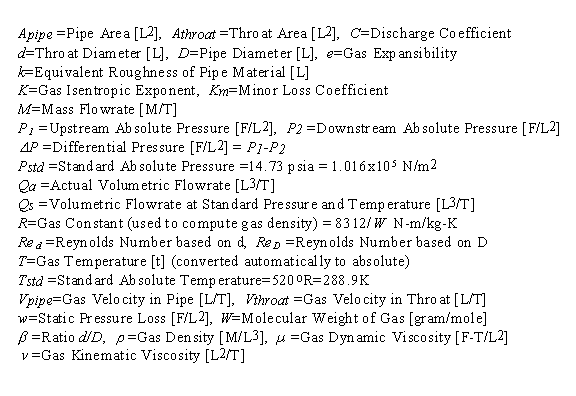Error Messages given by Orifice Calculation                             Top of Page
"P2/P1<0.8. Out of range". The equation for expansibility e is only valid for P2/P1≥0.8. This is a just a warning message; all variables are computed.

For the following error messages, only some variables are computed. For example if orifice throat diameter d is to be computed, then pressure ratio, expansibility, pipe area, pipe velocity, ReD, and some other variables will be computed and shown. However, if ReD is out of range for C to be valid, then C and d (and anything depending on d - such as throat area and throat velocity) will not be computed. If an error message is shown and you think your input is correct, be sure to check that you have selected the correct units for your entries.

"Infeasible input". While none of the inputs alone are out of range, they collectively result in a physically infeasible situation or a computed parameter will be out of range (e.g. ReD will be <1000 or d/D will be out of range) or the throat velocity will exceed the speed of sound (the orifice calculation is only valid for subsonic velocities).
"P1 and T (abs) must be >0", "Need P1 and T(abs)>0". Absolute pressure or absolute temperature was entered as zero or negative. If temperature was entered in C or F, it was internally converted to absolute temperature.

"Need 0.64<D<5 cm". Pipe diameter must be between 0.635 and 5.08 cm.
"Need 1e-20<Density<1e9 kg/m3". Gas density must be entered between 10-20 and 109 kg/m3.
"Need 1e-19<Viscosity<1e9 m2/s". Kinematic viscosity must be in this range. Note that kinematic viscosity is dynamic viscosity divided by density.
"Need 0.1<d/D<0.8". For orifice corner taps, diameter ratio must be in this range.
"Need 0.15<d/D<0.7". For orifice flange taps, diameter ratio must be in this range.
"Need K >1". Isentropic exponent was entered as ≤ 1.
"M or Q, and d must be >0". Mass flow rate, volumetric flow rates, and/or orifice diameter were entered as zero or negative.
"Need Δp > 0". Orifice differential pressure must be positive.
"Need Δp < P1". Orifice differential pressure cannot exceed P1; this would cause P2 (absolute) to be <0 which is impossible.
"M or Q, and Δp must be >0". Mass flow rate, volumetric flow rates, and/or differential pressure were entered as zero or negative
"Need ReD>1000". ReD must be at least 1000.

Try the simpler orifice calculation on our Bernoulli page if your parameters (for instance d/D, D, or ReD) are out of range. It is not as accurate, but won't give "parameter out of range" error messages.

References for Small Bore Orifice for Gas Flow Calculation                             Top of Page
American Society of Mechanical Engineers (ASME). 2001. Measurement of fluid flow using small bore precision orifice meters. ASME MFC-14M-2001.

Perry, R. H. and D. W. Green (editors). 1984. Perry's Chemical Engineers' Handbook. McGraw-Hill Book Co. 6th ed.

Weast, R. C. (editor). 1985. CRC Handbook of Chemistry and Physics. Chemical Rubber Company. 65th ed.

LMNO Engineering, Research, and Software, Ltd.
7860 Angel Ridge Rd.   Athens, Ohio 45701  USA   Phone: (740) 592-1890
LMNO@LMNOeng.com    http://www.LMNOeng.com

To:

Other flow meter calculations using standard methodologies:

Orifice for Gases (D>5cm)

Orifice for liquids (D<5cm)

Orifice for liquids (D>5cm)

Nozzle for liquids

Venturi for liquids

Simpler orifice calculation (not as accurate but won't give "parameter out of range" messages):

Bernoulli page

Pressure Relief Valve

Unit Conversions

Register